Take your time in this quiz!

# Pre-Algebra - Introduction to Radicals

This Math quiz is called 'Pre-Algebra - Introduction to Radicals' and it has been written by teachers to help you if you are studying the subject at middle school. Playing educational quizzes is a fabulous way to learn if you are in the 6th, 7th or 8th grade - aged 11 to 14.

It costs only \$12.50 per month to play this quiz and over 3,500 others that help you with your school work. You can subscribe on the page at Join Us

A radical is simply an expression that uses a root such as the square root of or the cube root of. There are three parts to a radical expression: (1) the radicand - which is simply the number you are trying to find the root of; (2) the radical symbol (√ - which means “root of”); and (3) the degree (or the number of times a number is multiplied by itself).

If you remember learning the “square root of” problems, when asked to find the square root of say “4”, the answer was “2”. This is because the square root of a number is a number times itself that will give you that beginning number.

As stated above, “4” is the beginning number and 2 times itself (i.e., 2 x 2) equals 4.

However, “2” is not the only square root of “4” as a “-2” times itself also equals “4” because a negative number times a negative number gives you a positive number. So, when asking what is the square root of “4,” the answer can be written as +, -2 (or the plus sign can be placed over the minus sign).

When asked to simplify the square root of 36 (√36), you are being asked to give the “positive” square root, not the negative. Sometimes this would be referred to as the “principal square root.”

When you are asked to give the square root of -√16, you are being asked to give the opposite or negative square root. For example, the square root of 16 is 4 but you are being asked for -√16 which would be -4.

Square root of variables: When finding the square root of a variable (an unknown number) you would have: √x2 = x because x times itself gives you x2. As another example: √8pq2 = 8pq.

Values: Real or Not - To determine if a value of a number is real, it must be a positive number. For example, √36 = 6 which is a real value. However, √-36 is not a real value because no number times itself will give you a negative 36. But, if you have -√36, the value would be a -6 because the question is asking for the opposite of the square root of 36. As the square root of 36 is 6, the opposite is -6.

For each numbered problem below, locate the correct answer. Be sure you pay attention to what the question is asking so you can find the correct answer.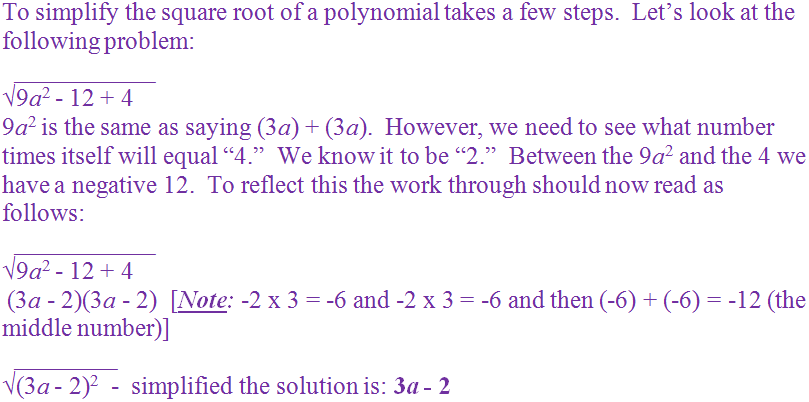Question 1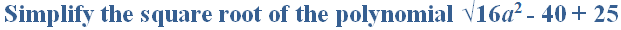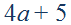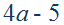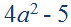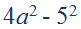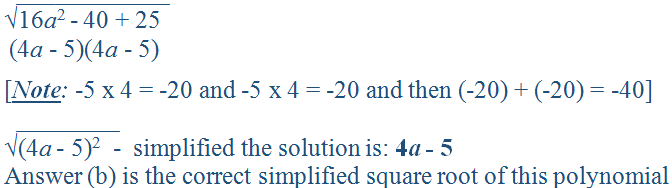Question 2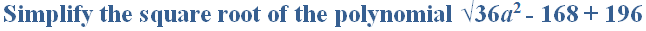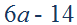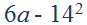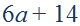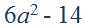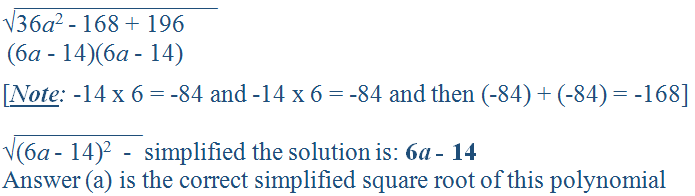Question 3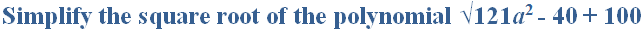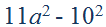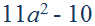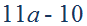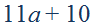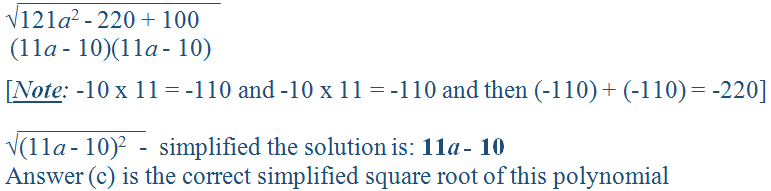Question 4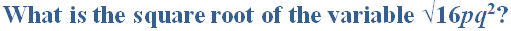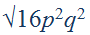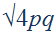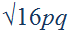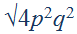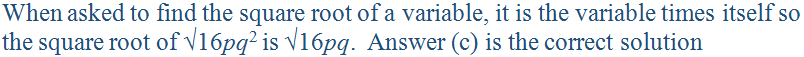Question 5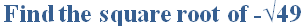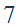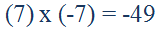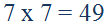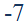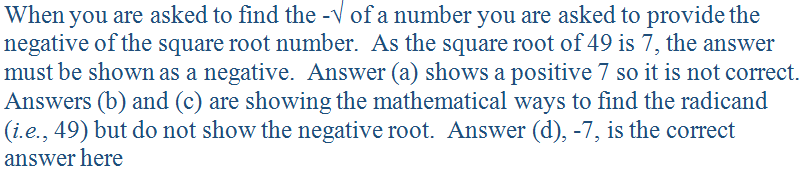Question 6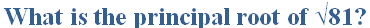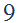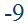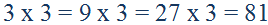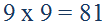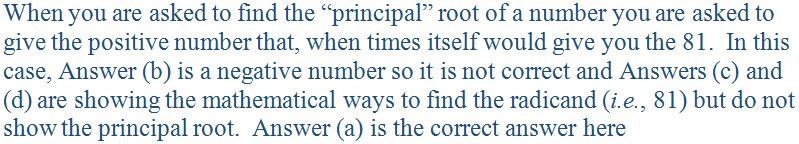Question 7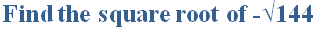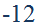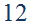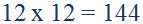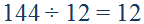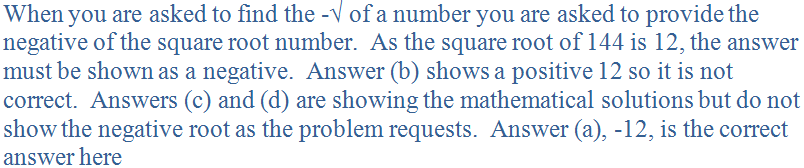Question 8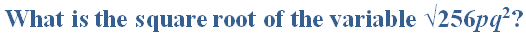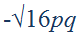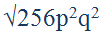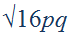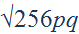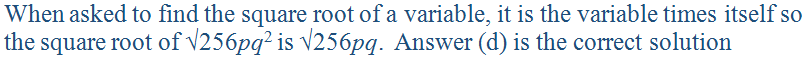Question 9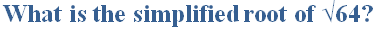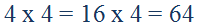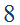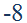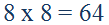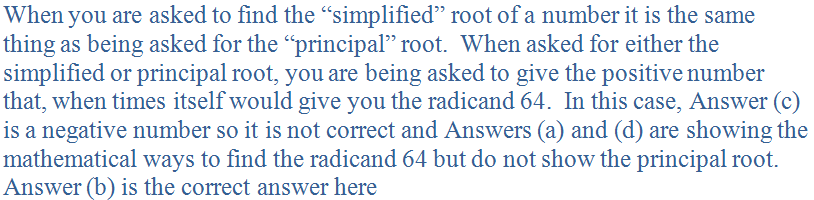Question 10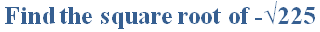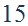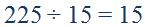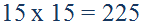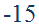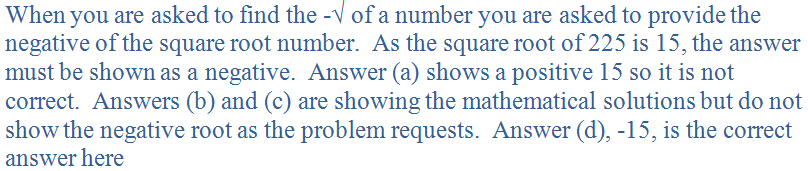Author:  Christine G. Broome